Next: 3.4 Carrier Mobility Up: 3.3 Band-Structure Previous: 3.3.4 Effective Carrier Mass

## 3.3.5 Effective Density of States

The effective density of states (DOS) in the conduction and the valence bands are expressed by the following theoretical expressions :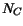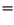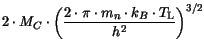(3.91)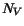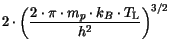(3.92)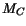represents the number of equivalent energy minima in the conduction band.

Table 3.19: Parameter values for energy minima in the DOS model
 MaterialMaterialSi 6 InAs 1 Ge 4 InP 1 GaAs 1 GaP 3 AlAs 3

For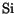an alternative model based on data after Green  is implemented, which is based on a second order polynomial fit.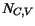(3.93)

Table 3.20: Parameter values for modeling the effective carrier masses
 Material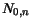[cm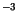]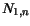[cm]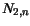[cm]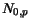[cm]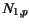[cm]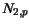[cm] Si -0.14e19 1.56e19 1.44e19 -0.17e19 0.93e19 2.34e19

In the model for alloy materials effective carrier masses of the constituents are used in the expressions (3.91) and (3.92).

In the case of a transition between a direct and an indirect bandgap in III-V ternary compounds the valley degeneracy factoris modeled by an expression equivalent to the one proposed in .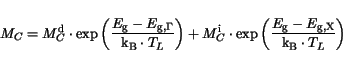(3.94)

The superscripts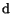anddenote direct and indirect, respectively.

In the case of SiGe the splitting of the valley degeneracy due to strain is modeled accordingly as in .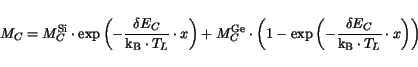(3.95)

Here,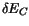denotes the energy difference between the valleys shifted down and up in energy, respectively. It is set equal to 0.6 eV as given in .Next: 3.4 Carrier Mobility Up: 3.3 Band-Structure Previous: 3.3.4 Effective Carrier Mass
Vassil Palankovski
2001-02-28